Karnataka Secondary Education Examination BoardKarnataka SSLC

# Right-angled Triangles and Pythagoras Property

#### definition

Right-Angled Triangles

Pythagoras Property

### Pythagoras Property:

(hypotenuse)2 = (base)2 + (height)2.

# 1. Right-Angled Triangles:

• Pythagoras, a Greek philosopher of the sixth century B.C. is said to have found a very important and useful property of right-angled triangles. The property is, hence, named after him.
• The Indian mathematician Baudhayan has also given an equivalent form of this property.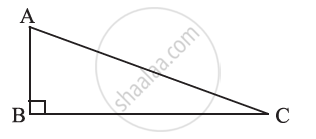• The side opposite to the right angle is called the hypotenuse; the other two sides are known as the legs of the right-angled triangle. In any right-angled triangle, the hypotenuse happens to be the longest side.
• In ∆ABC, the right-angle is at B. So, AC is the hypotenuse. bar"AB" and bar"BC" are the legs of ∆ABC.

# 2. Pythagoras Property:

(i) In a right-angled triangle, the square on the hypotenuse = sum of the squares on the legs.

From a card sheet, cut out eight identical right-angled triangles. Let us say the length of the hypotenuse of these triangles is ‘a’ units, and sides forming the right angle are ‘b’ and ‘c’ units. Note that the area of this triangle is "bc"/2. Next, on another card sheet, use a pencil to draw two squares ABCD and PQRS each of side (b + c) units. Now, place 4 of the triangle cut-outs in the square ABCD and the remaining 4 in the square PQRS as shown in the figures below. Mark by lines drawn across them, the parts of the squares covered by the triangles.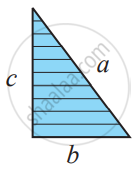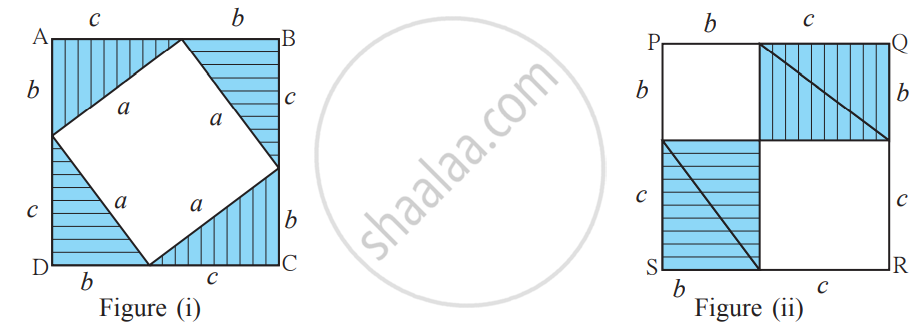Observe the figures. In figure (i) we can see a square of side a units in the uncovered portion of square ABCD. In figure (ii) we see a square of side b and another of side c in the uncovered portion of the square PQRS.

In figure (i), area of square ABCD = a2 + 4 × area of right-angled triangle

= a2 + 4 x 1/2bc

= a2 + 2bc

In figure (ii), area of square PQRS = b2 + c2 + 4 × area of right-angled triangle

= b2 + c2 + 4 × 1/2 bc
= b2 + c2 + 2bc

Area of square ABCD = Area of square PQRS
∴ a2 + 2bc = b2 + c2 + 2bc
∴ a2 = b2 + c2

(ii) Any right-angled triangle, the area of the square on the hypotenuse is equal to the sum of the areas of the squares on the legs.

Draw a right triangle, preferably on a square sheet, construct squares on its sides, compute the area of these square.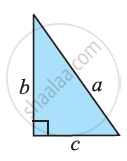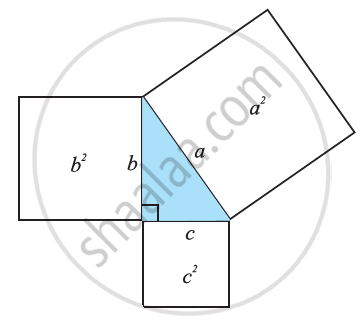a2 =b2 + c2

We will show that, if there is a triangle such that the sum of the squares on two of its sides is equal to the square of the third side, it must be a right-angled triangle.

If the Pythagoras property holds, the triangle must be right-angled.

Pythagoras property says that for any right-angled triangle, the area of the square on the hypotenuse is equal to the sum of the areas of the squares on the legs.

#### Example

Determine whether the triangle whose lengths of sides are 3 cm, 4 cm, 5 cm is a right-angled triangle.

32 = 3 × 3 = 9;
42 = 4 × 4 = 16;
52 = 5 × 5 = 25

We find 32 + 42 = 52.
Therefore, the triangle is right-angled.

#### Example

∆ABC is right-angled at C. If AC = 5 cm and BC = 12 cm. find the length of AB.

A rough figure will help us.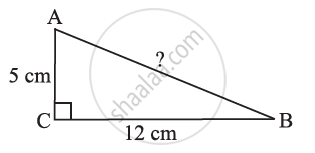By Pythagoras property,

AB2 = AC2 + BC2
AB2 = 52 + 122

AB2 = 25 + 144

AB2 = 169

AB2 = 132

AB2 =13.

So, AB = 13 or the length of AB is 13 cm.

If you would like to contribute notes or other learning material, please submit them using the button below.

#### Video Tutorials

We have provided more than 1 series of video tutorials for some topics to help you get a better understanding of the topic.

Series 1

Series 2

### Shaalaa.com

Triangles part 39 (Proof Converse of Pythagoras Theorem) [00:03:31]
S